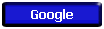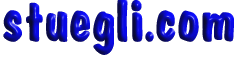# Earth Orbital VelocityThe velocity of a satellite in circular orbit around the Earth depends upon the radius of the orbit and the acceleration of gravity at the orbit. Above the earth's surface at a height of h =m = x 106 m, which corresponds to a radius r = x earth radius, the acceleration of gravity is g = m/s2 = x g on the earth's surface. At the specified orbit radius, the required orbit velocity is v =m/s=km/h=mi/h. The period of the orbit is minutes = hours.Copyright © 2005 -  S. B. EglICopyright © 2005 -  S. B. EglI